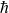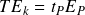# Planck’s constant

Planck’s constant is usually associated with quantum mechanics since it is used to calculate physical phenomena on tiny scales. However, the elementary form of Planck’s constant and the gravitational constant show that either constant could be used for quantum or gravitational systems if we toss in the right mix of Planck units. The relationship between the two constants isPlanck’s constant is simply a convenient mixtures of Planck units for building the right operators and potentials required by a given formula. Planck’s constant includes the product of Planck length and Planck mass () making it suitable for calculating the extensive properties of elementary particles.

The elementary form of Planck’s constant is

Planck’s constant is sometimes called the “quantum of action” because it has the unit dimensions of action in both length x momentum and energy x time. Each of the following forms is an equivalent depiction of Planck’s constant

## Conservation

New Foundations Model symmetries show that mechanical properties of elementary particles conserve Planck’s constant, as demonstrated for photons and electrons.

Because Planck’s constant is conserved, it has been said that angular momentum is quantized in units of, but this is a poor characterization. Planck’s constant is not a fundamental unit but a conserved quantity for all combinations of particle wavelength, inertial mass, and momentum according to the formula.

The constant is also conserved for all combinations of oscillation period and kinetic energy.

## Planck’s constant in historical formulas

Planck’s constant is versatile because its mixture of Planck units can be assembled into a number of different formulas. Most notably, the ratio of Planck’s constant to a particle’s wavelength creates the adaptable wavelength operator which quantifies inertial mass and momentum. The wavelength operator transforms the Planck mass to produce inertial mass in dimension M, or the Planck momentum to produce momentum in dimensions LMT-1. The following illustration shows how the traditional formulatransforms into the correct operators and potential for finding a particle’s momentum.

Calculating the energy of a photon requires an additional quantity ofin the numerator and the same wavelength operator will transform the Planck energy into the correct energy of a photon. No additional operators are needed because photons move at the speed of light and are spin 1 particles.

Planck’s constant is also used to find a particle’s wavelength from a quantity of rest mass. Inputs of rest mass and velocity create two operators–one producing the corresponding wavelength of the given mass, and a second that has no affect on the wavelength because the particle is moving at the speed of light.

For particles moving at less than light speed, Planck’s constant finds the wavelength using the same two operators, but this time rest mass and velocity transform the wavelength.

Planck’s constant